Ex 12.2

Chapter 12 Class 12 Linear Programming
Serial order wise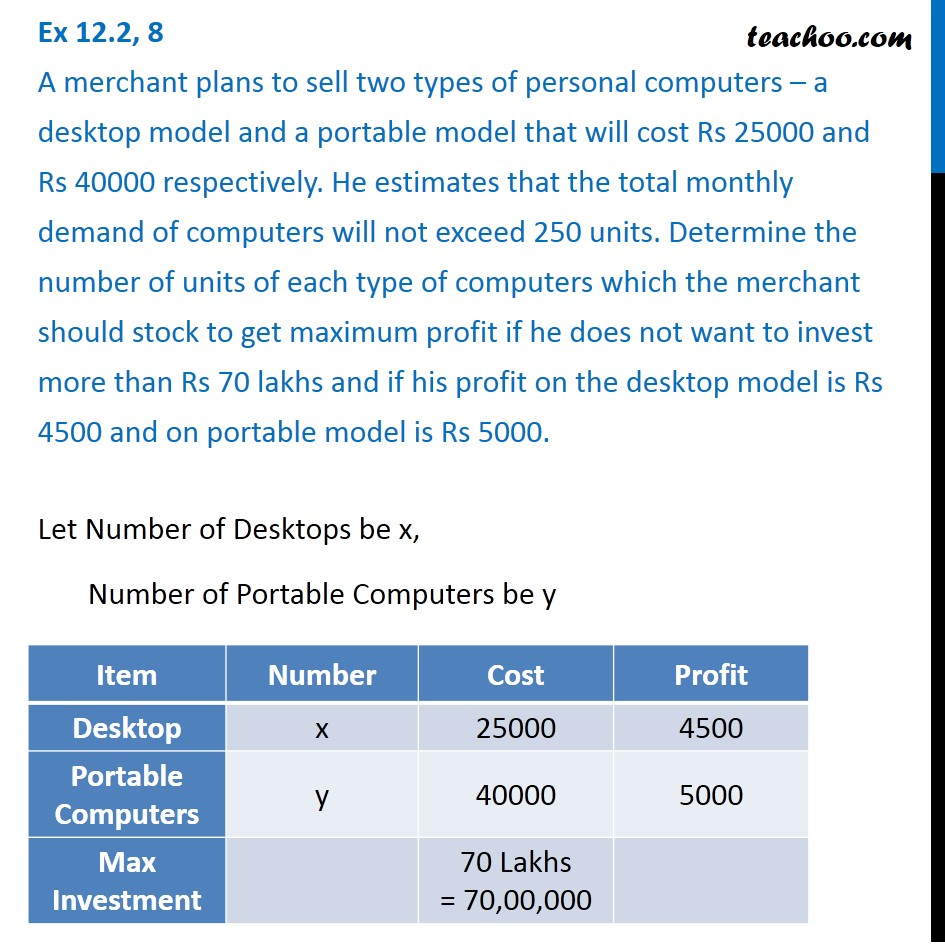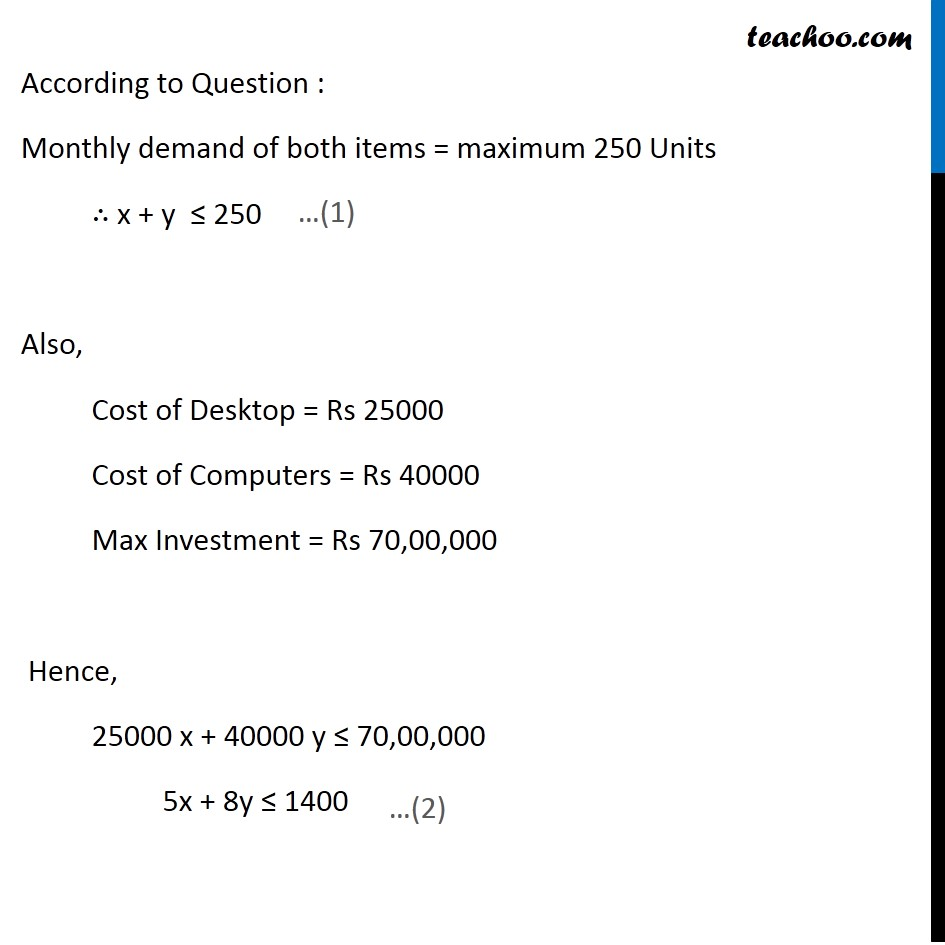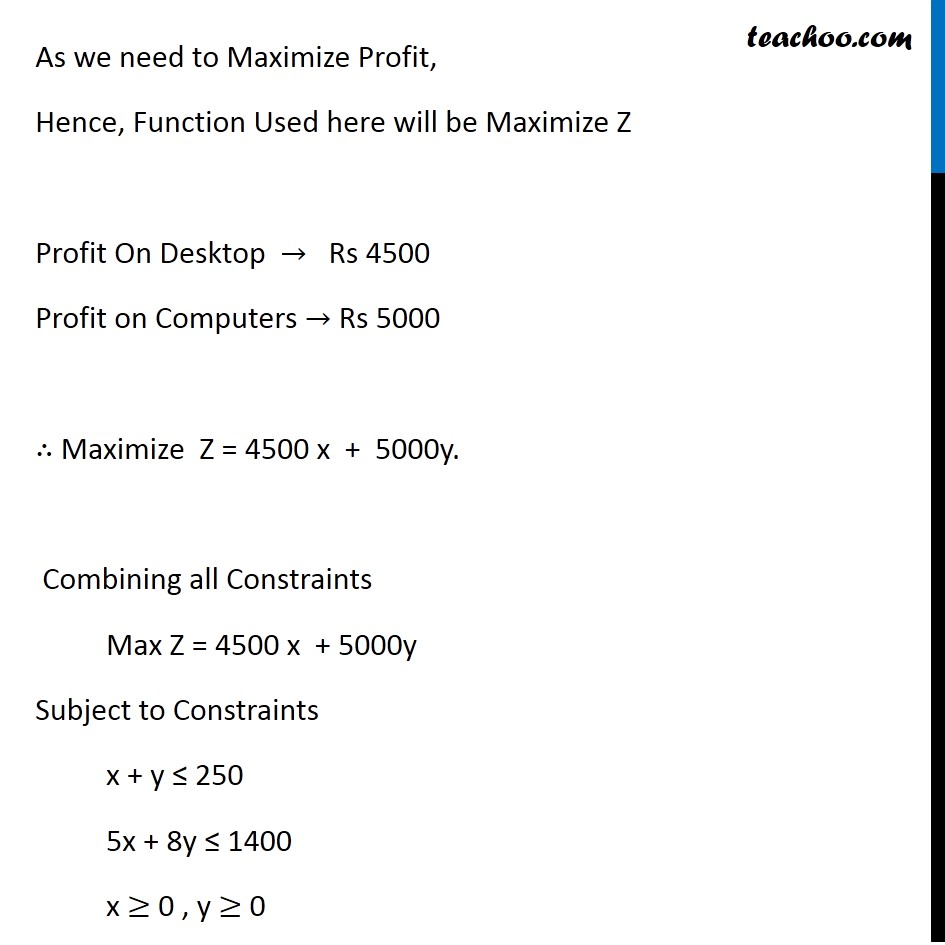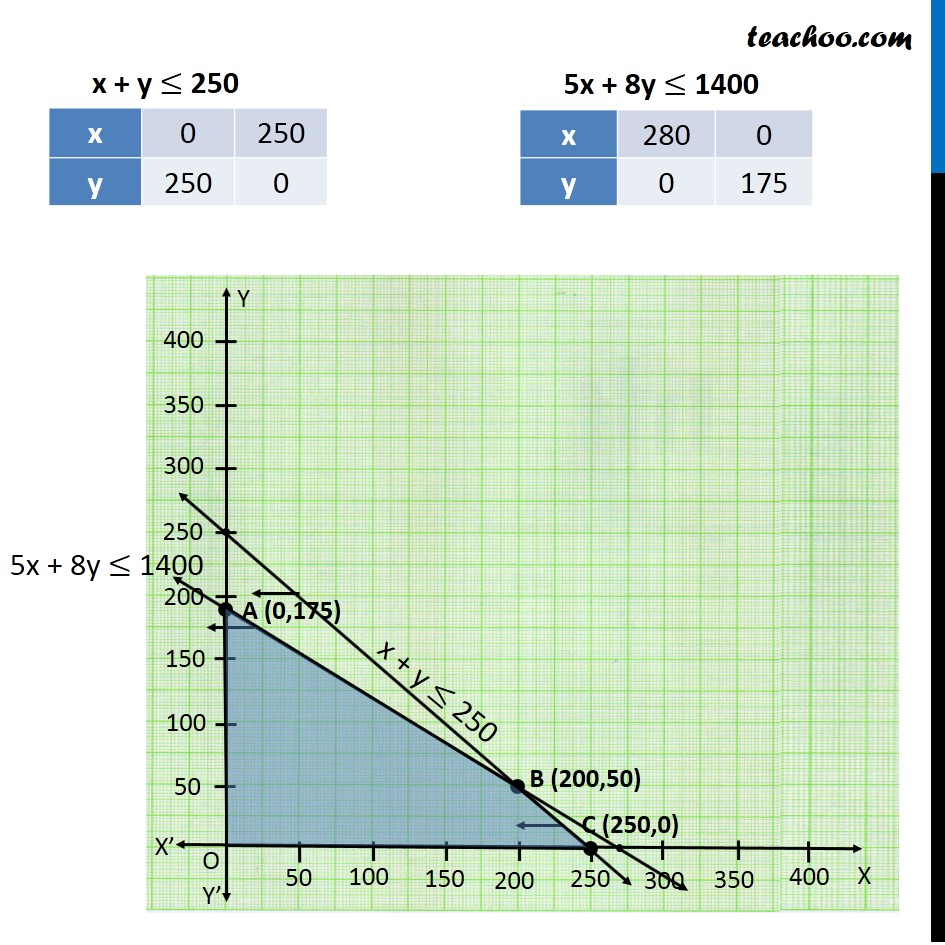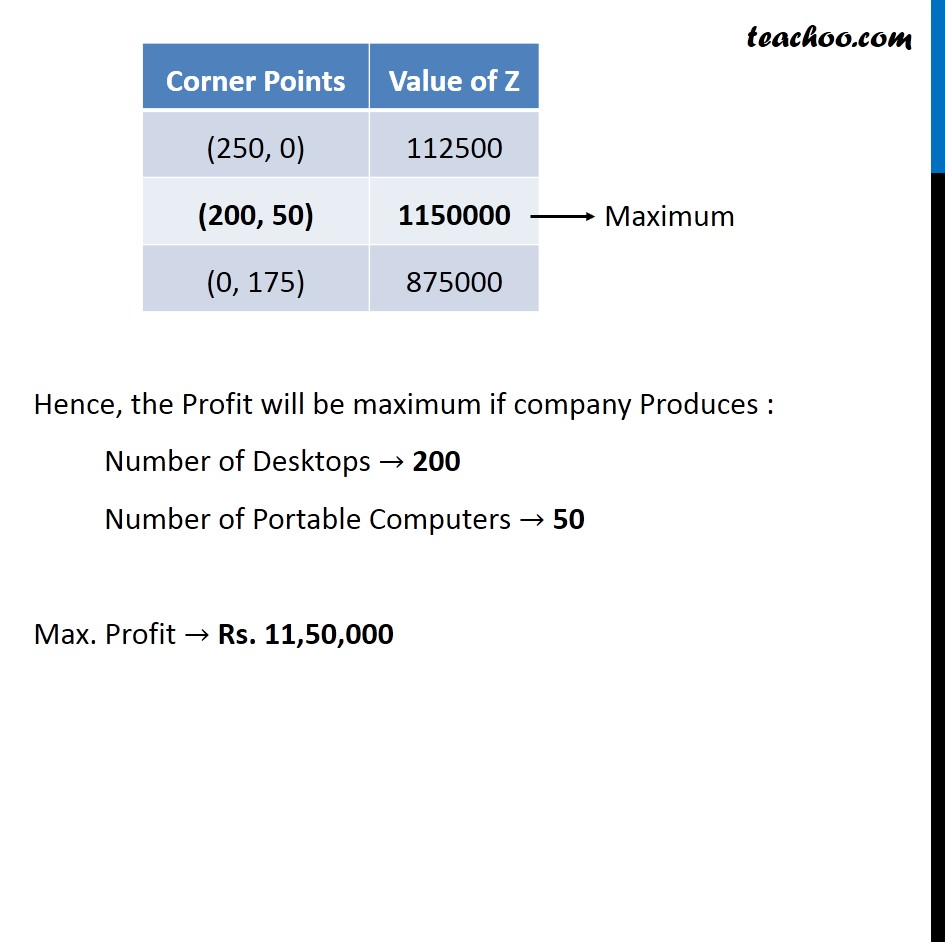Get live Maths 1-on-1 Classs - Class 6 to 12

### Transcript

Ex 12.2, 8 A merchant plans to sell two types of personal computers – a desktop model and a portable model that will cost Rs 25000 and Rs 40000 respectively. He estimates that the total monthly demand of computers will not exceed 250 units. Determine the number of units of each type of computers which the merchant should stock to get maximum profit if he does not want to invest more than Rs 70 lakhs and if his profit on the desktop model is Rs 4500 and on portable model is Rs 5000. Let Number of Desktops be x, Number of Portable Computers be y According to Question : Monthly demand of both items = maximum 250 Units ∴ x + y ≤ 250 Also, Cost of Desktop = Rs 25000 Cost of Computers = Rs 40000 Max Investment = Rs 70,00,000 Hence, 25000 x + 40000 y ≤ 70,00,000 5x + 8y ≤ 1400 As we need to Maximize Profit, Hence, Function Used here will be Maximize Z Profit On Desktop → Rs 4500 Profit on Computers → Rs 5000 ∴ Maximize Z = 4500 x + 5000y. Combining all Constraints Max Z = 4500 x + 5000y Subject to Constraints x + y ≤ 250 5x + 8y ≤ 1400 x ≥ 0 , y ≥ 0 Hence, the Profit will be maximum if company Produces : Number of Desktops → 200 Number of Portable Computers → 50 Max. Profit → Rs. 11,50,000